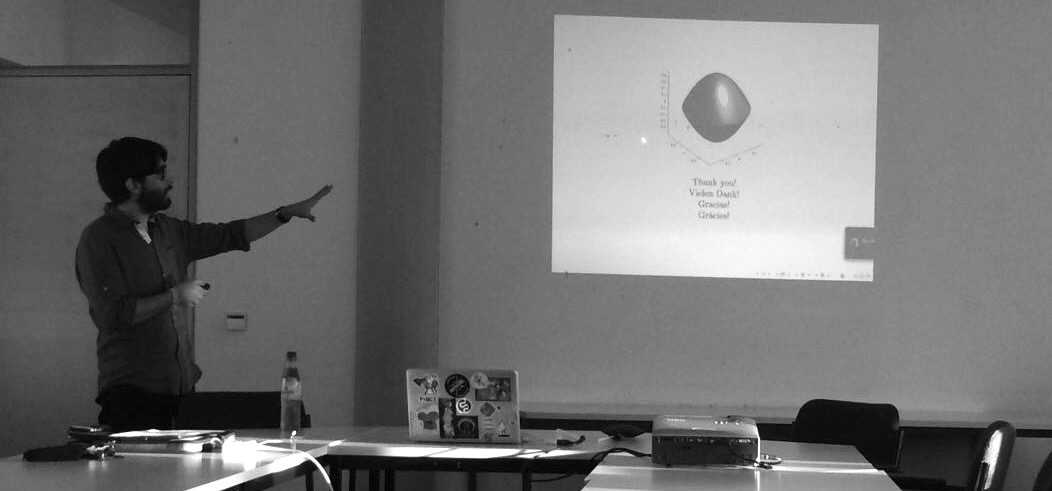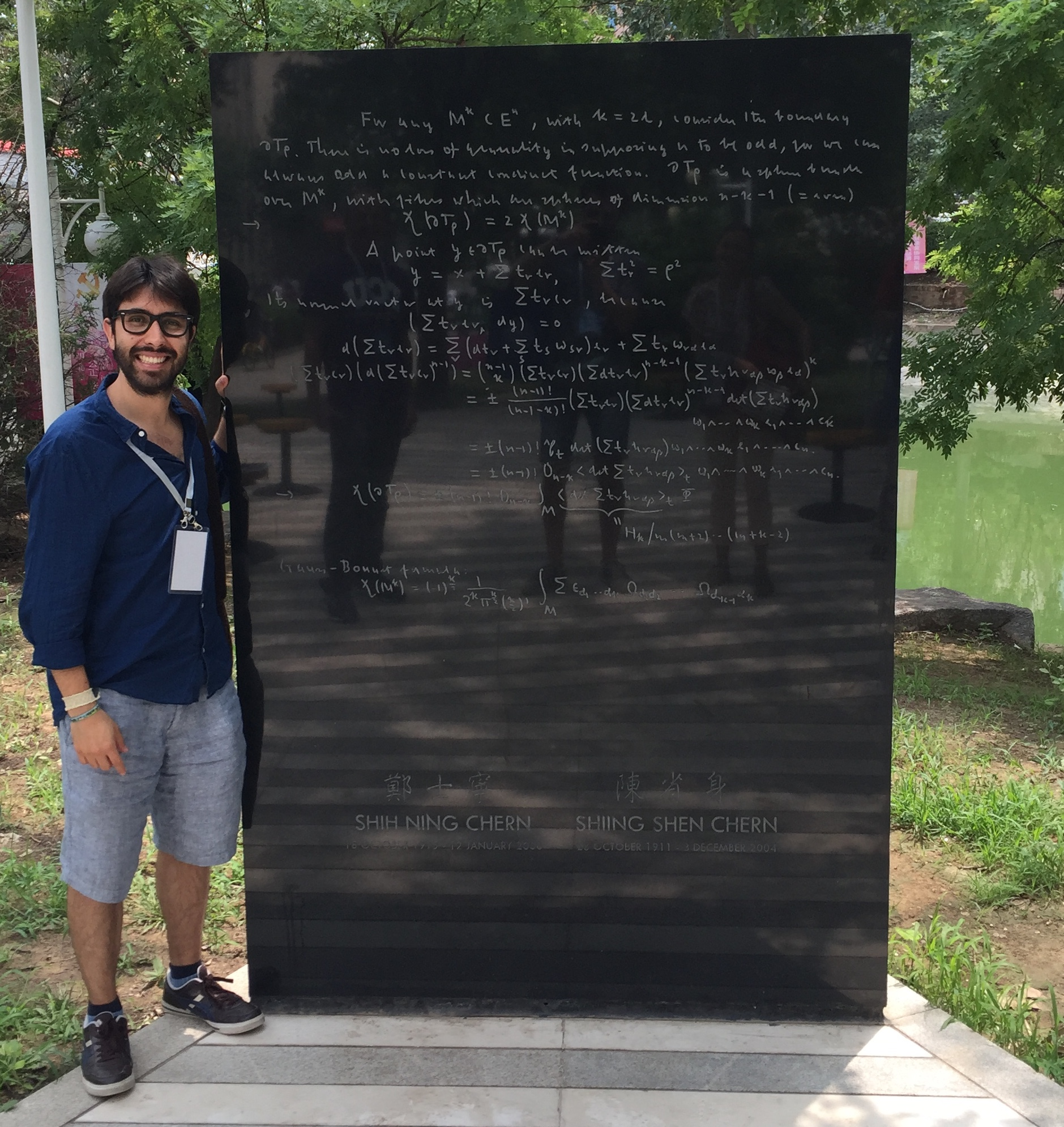# Induced Dirac-Schrödinger operators on semi-free circle quotients

In this post I present the results of my Mathematics PhD thesis which I wrote under the supervision of Prof. Dr. Jochen Brüning at Humbolt Universität zu Berlin. I gratefully acknowledge the financial support of the Berlin Mathematical School and the research project SFB 647: Space - Time - Matter

Abstract:

John Lott has computed an integer-valued signature for the orbit space of a compact orientable $$(4k+1)$$ manifold with a semi-free $$S^1$$-action, which is a homotopy invariant of that space, but he did not construct a Dirac type operator which has this signature as its index. In this Thesis, we construct such operator on the orbit space, a Thom-Mather stratified space with one singular stratum of positive dimension, and we show that it is essentially unique and that its index coincides with Lott’s signature, at least when the stratified space satisfies the so called Witt condition. We call this operator the induced Dirac-Schrödinger operator. The strategy of the construction is to “push down” an appropriate $$S^1$$-invariant first order transversally elliptic operator to the quotient space.

The Witt condition, a topological condition which in this case depends on the codimension of the fixed point set, has various analytic consequences. In particular, when not satisfied, the Hodge-de Rham operator on the quotient space does not need to be essentially self-adjoint and therefore a choice of boundary conditions is required. This choice freedom is not natural in view of the fact that Lott’s signature is well defined independently of the Witt condition.

The Dirac-Schrödinger operator constructed in this Thesis differs from the Hodge-de Rham operator by a zero order term which ensures it to be essentially self-adjoint. Moreover, this zero order term anti-commutes with the chirality involution allowing the whole operator to split so that the index can be computed even if the Witt condition is not satisfied.This picture was taken at the end of my thesis seminar at Humboldt Universität zu Berlin.This picture was taken at the Chern Institute of Mathematics in Tianjin (China) where I had the oportunity to present the results of this thesis. Here I am with Chern’s proof of the Chern-Gauß-Bonnet Theorem.